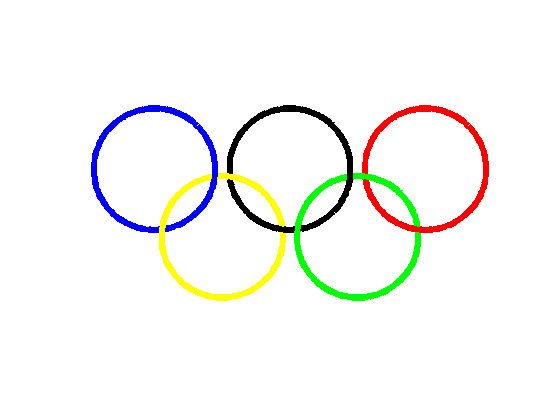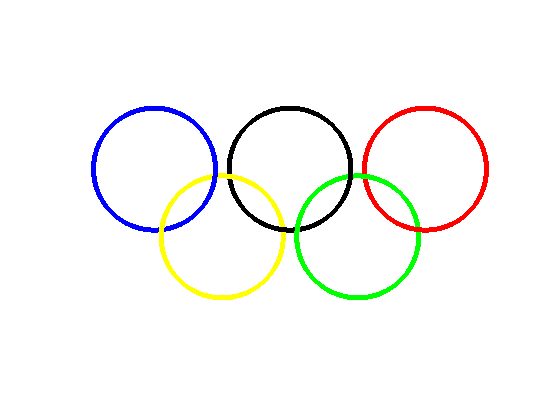## Loren on the Art of MATLABTurn ideas into MATLAB

Note

Loren on the Art of MATLAB has been retired and will not be updated.

# Olympic Rings

Well, I am very late on this challenge. In August, Mike posted some code to reproduce the Olympic rings and mentioned that I might have a more clever way to create the same plot. Here's my attempt.

### Create X and Y Values for the 5 Rings

First, I'll follow Mike's parameters and code to create the initial five rings.

N = 1000;
angle = linspace(pi/4,9*pi/4,N);

xb = cos(angle) * 0.9;
yb = sin(angle) * 0.9;

xy = cos(angle) * 0.9 + 1;
yy = sin(angle) * 0.9 - 1;

xk = cos(angle) * 0.9 + 2;
yk = sin(angle) * 0.9;

xg = cos(angle) * 0.9 + 3;
yg = sin(angle) * 0.9 - 1;

xr = cos(angle) * 0.9 + 4;
yr = sin(angle) * 0.9;

### Mike's Rings

What Mike does next is to break rings into segments for plotting so the last ring plotted in any given location is the color that should be on top.

h1 = figure;
hold on
plot(xb(1:3*N/4),yb(1:3*N/4),'b','linewidth',5);
plot(xy(N/4:N),yy(N/4:N),'y','linewidth',5)

plot(xk(1:3*N/4),yk(1:3*N/4),'k','linewidth',5);
plot(xy(1:N/4),yy(1:N/4),'y','linewidth',5);
plot(xb(3*N/4:end),yb(3*N/4:end),'b','linewidth',5);

plot(xr(1:N/2),yr(1:N/2),'r','linewidth',5);
plot(xg(1:N),yg(1:N),'g','linewidth',5);

plot(xk(3*N/4:N),yk(3*N/4:N),'k','linewidth',5);
plot(xr(N/2:N),yr(N/2:N),'r','linewidth',5);

% make the axis pretty
axis equal
axis off
xlim([-1.2 5.2])
set(h1,'Color',[1 1 1])
hold off### My Solution

My "cleverness" is to offset the rings in the Z-plane.

First I noticed that all the top rings have an axis about which I can "tilt" them ever so slightly to get the most of the over/under behavior for the rings. Add small Z values to each ring.

thetab = -pi/4;
thetak = -pi/4;
thetar = -pi/4;

Now I create Z values for the rings in the upper rows by tilting them about the axes defined by the angles above (which happen to be identical).

zb = cos(angle + thetab) * 0.1;
zk = cos(angle + thetak) * 0.1;
zr = cos(angle + thetar) * 0.1;

Next I deal with the lower rings in a lazy way, introducing a discontinuity in Z. If I had more patience today, I could have come up with an undulation in Z that was more than a tilted plane or a step.

I find the angles where I need to depress the bottom rings relative to the top and set appropriate Z values for these to be less than 0.

mask = angle >= 2*pi;
zg = zeros(size(xb));
zy = zeros(size(xb));
zy(mask) = -0.1;

### Loren's Rings

Here's my rendition of the rings. My plot commands are a bit less complicated than Mike's. I do the work earlier by setting Z values. Also, because of my laziness, if you were to rotate my version of rings in 3-D, you'd see the bottom two aren't really rings, but have discontinuities.

h2 = figure;
hold on
plot3(xb,yb,zb,'b.','markersize',10);
plot3(xy,yy,zy,'y.','markersize',10);
plot3(xk,yk,zk,'k.','markersize',10);
plot3(xg,yg,zg,'g.','markersize',10);
plot3(xr,yr,zr,'r.','markersize',10);
% make the axis pretty
axis equal
axis off
xlim([-1.2 5.2])
set(h2,'Color',[1 1 1])
hold off### Any Other Methods?

Mike and I show 2 methods to generate interlocking rings. Do you have any other ideas for doing this? Let me know here.

Published with MATLAB® 7.7

|